• # Math

Browse All 17 ResourcesIn this activity, students explore the importance of charts to navigation on bodies of water. Using one worksheet, students learn to read the major map features found on a real nau...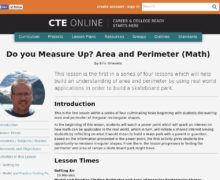This lesson is the first in a series of four lessons which will help build an understanding of area and perimeter by using real world applications in order to build a skateboard pa...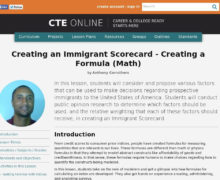In this lesson, students will consider and propose various factors that can be used to make decisions regarding prospective immigrants to the United States of America. Students wil...Juxtaposing the architecture of Ellis island with that of the proposed Trump Wall creates many opportunities for mathematical analysis and frames a discussion of how our nation's a...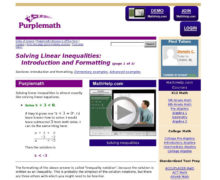From Elizabeth Stapel at Purplemath, this module helps students understand how to solve linear inequalities with an introduction to the topic using both inequality notation and a g...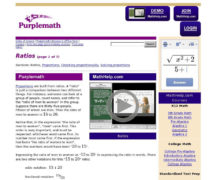This set of instructional materials and problems helps students understand ratios and proportions, starting with a simple review and progressing into the more advanced territory of...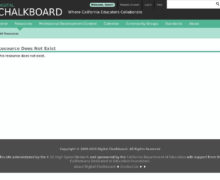Students will see an animated presentation of the proof of the Pythagorean Theorem. This animated PowerPoint presentation uses shearing and the invariance of the area of triangles ...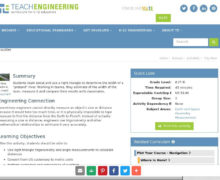Students learn about and use a right triangle to determine the width of a "pretend" river. Working in teams, they estimate of the width of the river, measure it and compare their r...In this tutorial, "Linear functions of the form f(x) = a x + b and the properties of their graphs are explored interactively using an applet." The applet allows students to manipul...These 20 images give students practice measuring the height and width of various pictures with paperclips. Ready to print, each sheet contains a spot for students to write in their...The classroom poster helps students understand fractions as parts of a whole by illustrating pizzas and fractions of pizzas. These eight images show a whole pizza, a pizza cut in h...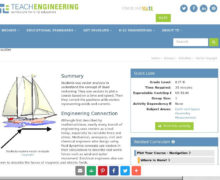Students use vector analysis to understand the concept of dead reckoning. They use vectors to plot a course based on a time and speed. Then they correct the positions with vectors ...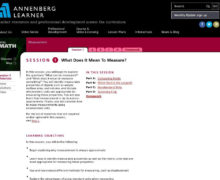In this session, students will begin to explore the questions "What can be measured?" and "What does it mean to measure something?" Students identify measurable properties of objec...When it comes to teaching students how to make sure your foundation has a true 90 degree corner to work from, I like to use the simple "3, 4, 5 Rule." This is also known as the Pyt...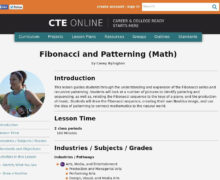This lesson guides students through the understanding and expansion of the Fibonacci series and recursive patterning. Students will look at a number of pictures to identify patteri...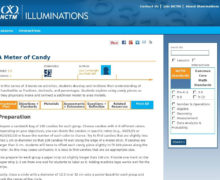Developing a sense of hundredths through the use of models In this series of 3 hands-on activities, students develop and reinforce their understanding of hundredths as fractions, d...